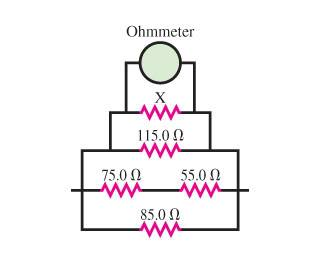# Resistors and Ohmmeter

Soaring Crane

## Homework Statement

For the resistor network shown in the figure, the ohmmeter reads 20.2 ohm.What is the resistance of the resistor X?

See below.

## The Attempt at a Solution

I’m uncertain if I tackled the system correctly, so please guide me on any misconceptions in my workings.

For an ohmmeter, I didn’t come across any special formulas like for a voltmeter, and I may have not addressed any typical properties for ohmmeter readings . . .

Are the 75.0 ohm and 55.0 ohm in series? And then X; R_75+55; R_115, and R_85 will be in parallel?

(1/R_eq) = (1/R_x) +(1/115.0 ohm) +(1/130.0 ohm) + (1/85 ohm)
(1/R_x) = (1/20.2 ohm) – (1/115.0 ohm) –(1/130 ohm) – (1/85 ohm)
= 0.02135 1/ohm

R_x = 1/0.0214 1/ohm = 46.8 ohm ???

Thanks.